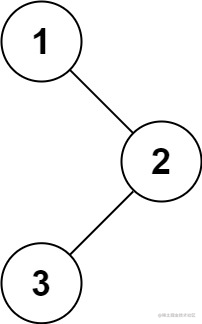# 题目• 输入：`root = [1,null,2,3]`
• 输出：`[3,2,1]`

• 输入：`root = []`
• 输出：`[]`

• 输入：`root = `
• 输出：``

# 方法一：递归

## 代码

``````class Solution {
func preorder(_ root: TreeNode?, _ res: inout [Int]) {
guard nil != root else {
return
}
preorder(root?.left, &res)
preorder(root?.right, &res)
res.append(root!.val)
}

func postorderTraversal(_ root: TreeNode?) -> [Int] {
var res: [Int] = []
preorder(root, &res)
return res
}
}

## 复杂度分析

• 时间复杂度：O(n)，其中 n 是二叉树的节点数。每一个节点恰好被遍历一次。

• 空间复杂度：O(n)，为递归过程中栈的开销，平均情况下为 O(log n)，最坏情况下树呈现链状，为 O(n)。

# 方法二：迭代

## 代码

``````class Solution {
func postorderTraversal(_ root: TreeNode?) -> [Int] {
var res: [Int] = []
if nil == root {
return res
}
var stack: [TreeNode] = []
var node: TreeNode? = root
var prev: TreeNode? = nil

while !stack.isEmpty || nil != node {
while nil != node {
stack.append(node!)
node = node?.left
}
node = stack.popLast()
if nil == node?.right || node?.right == prev {
res.append(node!.val)
prev = node
node = nil
} else {
stack.append(node!)
node = node?.right
}
}
return res
}
}

extension TreeNode: Equatable {
public static func == (lhs: TreeNode, rhs: TreeNode) -> Bool {
return lhs === rhs
}
}

## 复杂度分析

• 时间复杂度：O(n)，其中 n 是二叉树的节点数。每一个节点恰好被遍历一次。

• 空间复杂度：O(n)，为迭代过程中显式栈的开销，平均情况下为 O(log n)，最坏情况下树呈现链状，为 O(n)。

# 方法三：Morris 遍历

## 思路及解法

1. 新建临时节点，令该节点为 `root`
2. 如果当前节点的左子节点为空，则遍历当前节点的右子节点；
3. 如果当前节点的左子节点不为空，在当前节点的左子树中找到当前节点在中序遍历下的前驱节点；
• 如果前驱节点的右子节点为空，将前驱节点的右子节点设置为当前节点，当前节点更新为当前节点的左子节点。
• 如果前驱节点的右子节点为当前节点，将它的右子节点重新设为空。倒序输出从当前节点的左子节点到该前驱节点这条路径上的所有节点。当前节点更新为当前节点的右子节点。
4. 重复步骤 2 和步骤 3，直到遍历结束。

## 代码

``````class Solution {
func postorderTraversal(_ root: TreeNode?) -> [Int] {
var res: [Int] = []
if nil == root {
return res
}

var p1: TreeNode? = root
var p2: TreeNode? = nil
while (nil != p1) {
p2 = p1?.left
if (nil != p2) {
while (nil != p2?.right && p2?.right != p1) {
p2 = p2?.right
}
if (nil == p2?.right) {
p2?.right = p1
p1 = p1?.left
continue
} else {
p2?.right = nil
}
}
p1 = p1?.right
}
var tempNode: TreeNode? = root
return res
}

func addPath(_ res: inout [Int], _ node: inout TreeNode?) {
var count: Int = 0
while (nil != node) {
count += 1
res.append(node!.val)
node = node?.right
}
var leftCount = res.count - count
var rightCount = res.count - 1
while (leftCount < rightCount) {
let temp: Int = res[leftCount]
res[leftCount] = res[rightCount]
res[rightCount] = temp
leftCount += 1
rightCount -= 1
}
}
}

extension TreeNode: Equatable {
public static func == (lhs: TreeNode, rhs: TreeNode) -> Bool {
return lhs === rhs
}
}

## 复杂度分析

• 时间复杂度：O(n)，其中 n 是二叉树的节点数。没有左子树的节点只被访问一次，有左子树的节点被访问两次。

• 空间复杂度：O(1)。只操作已经存在的指针（树的空闲指针），因此只需要常数的额外空间。

iOS